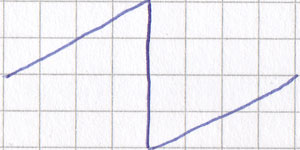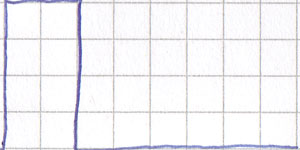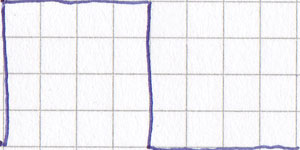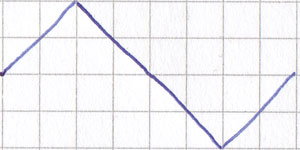# ByteNoise

## Periodic waveform

A periodic waveform is one which repeats the exact same shape again and again. It doesn't change, so both its shape and its harmonic content stay the same for the waveform's whole duration.

There are four main periodic waveforms used in synthesis: the sine wave, sawtooth wave, pulse wave and triangle wave.

### Harmonics

Before I go any further, it's probably best to explain just what "harmonic content" means. A waveform can be thought of as many different sine waves, the slowest of which is the frequency of the note. The shape of the waveform is determined by how loud the other sine waves are.

That slowest sine wave is called the fundamental harmonic. In musically pleasant timbres, the other sine waves present in the waveform are multiples of the fundamental harmonic. So there may be a sine wave that's twice as fast as the fundamental harmonic, and there may be one that's three times its speed, and one that's four times its speed, and so on.

Each of these sine waves is called a harmonic. The slowest one is the fundamental harmonic, the one twice its speed is the second harmonic, the one three times its speed is the third harmonic, and so on.

The harmonic content of a waveform, then, is another way of saying which frequencies of sine waves are present, and how loud they are. While you probably don't need to know this, it will hopefully let you look at the same thing in different ways, and give you a better understanding of how things such as filters work.

### Sine wave

As all sounds are made up of sine waves, it makes sense that one sine wave in isolation doesn't give you much to play with. You need to play it pretty loudly to hear it, but it will sound powerful if played loud enough and at a low enough frequency (which is the basis for the Roland TR-808's ubiquitous kick drum). Filtering a sine wave is pointless, as the whole purpose of a filter is to boost or quieten certain frequencies — certain sine waves present in the original waveform — so all a filter can do to a single sine wave is make it louder or quieter, which is no better than running it through an attenuator or amplifier instead.

A single sine wave in isolation is mainly used as a strange, ethereal sound (like a Theremin might create), or for a strong, clean bass sound (assuming the listener has good enough speakers to actually hear it).

### Sawtooth waveA sawtooth wave

As its name suggests, the sawtooth wave resembles the teeth of a saw. There are two kinds of sawtooth wave: the ramp wave, which slowly rises then quickly falls, is the more common of the two, but a slowly falling sawtooth wave is also possible to create. They sound exactly the same, though, so there's rarely a point in creating both (the main reasons are to get them to cancel each other out in interesting ways, or to modify other sounds).

### Ramp wave

The ramp wave, otherwise known as the rising sawtooth wave, is very rich because it includes all harmonics. This means it includes the fundamental harmonic, and another sine wave at twice its speed, and another at three times its speed, and so on. The second sine wave is half as loud, as well as twice as fast. The third one is a third as loud, as well as three times the speed. The mathematical way of saying this is that each harmonic (each sine wave present in the waveform) is 1/n (its volume is the volume of the fundamental harmonic, divided by the number of the harmonic).

What this means in practical terms is that the ramp waveform is a rich timbre that provides a filter with plenty of harmonics to work with. This makes it a good starting point for a patch.

### Pulse waveA pulse wave with a duty cycle of 25%

The pulse wave has only two possible states: on or off. The most often used pulse wave is the square wave, which spends exactly the same amount of time in the "on" position as it does in the "off" position.

The amount of time a pulse wave spends in the "on" position is called its duty cycle. A pulse wave which spends a quarter of the time on, then three quarters off before repeating, is said to have a 25% duty cycle.

The duty cycle dictates the timbre of the sound. A pulse wave with a 1% duty cycle sounds different from a pulse wave with a 50% duty cycle. This is because they are different shapes, and therefore have different harmonic contents. Once the halfway mark is reached, though, the sounds repeat — a 55% duty cycle sounds the same as a 45% one, and a 66% duty cycle sounds the same as a 33% one. For this reason, many oscillators that output pulse waves only let you set a duty cycle of between about 1% and 50%. A duty cycle of exactly 0% or 100% would be completely quiet, as the waveform would just be a straight line, and sound is only made when something moves.

Changing the duty cycle over time creates an evolving timbre — a quasi-periodic waveform instead of a periodic one. This is called pulse width modulation.

### Square waveA square wave

The square wave is a pulse wave with a duty cycle of 50%. It only has odd harmonics (the first sine wave, the third one, the fifth and so on), but they are still 1/n where n is the number of the harmonic. So a square wave is like a ramp wave, only with every even numbered harmonic missing. This makes it sound hollow in comparison.

### Triangle waveA triangle wave

A triangle wave looks a bit like a sawtooth wave, only without the vertical lines. It slowly falls then slowly rises, making it look like a zigzag shape.

Again, only odd harmonics are present in the triangle wave. The amplitude of each harmonic, however, is 1/n2. This causes the higher harmonics to be much quieter than they are in a square wave. For this reason, the triangle wave can be a good starting point for a bass instrument.

It is possible to create just about any shape you can think of in a waveform (bearing in mind that the speaker cone can only be in one position at any given time) and loop it to create a periodic waveform. The ones listed above are just the periodic waveforms most often produced by the oscillators (both audible and low frequency oscillators) of synthesisers, and also the simplest to describe mathematically and harmonically.

The human ear gets bored of harmonic waveforms pretty quickly, though, as they are too predictable. This is possibly why some people swear by unstable analogue circuitry to produce such waveforms. It is also where synthesis can be taken to the next step: quasi-periodic waveforms.